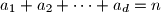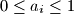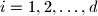### IMO Shortlist 2013 problem C1

Kvaliteta:
Avg: 4,0
Težina:
Avg: 5,5
Let$n$ be a positive integer. Find the smallest integer$k$ with the following property: Given any real numbers$a_1, \ldots, a_d$ such that$a_1 + a_2 + \cdots + a_d = n$ and$0 \leq a_i \leq 1$ for$i = 1, 2, \ldots, d$, it is possible to partition these numbers into$k$ groups (some of which may be empty) such that the sum of the numbers in each group is at most$1$.
Izvor: Poland